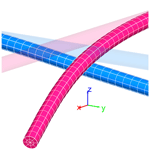https://doi.org/10.5194/ms-9-373-2018
https://doi.org/10.5194/ms-9-373-2018# Rotational Constraint between Beams in 3-D Space

Hamid Reza Motamedian and Artem Kulachenko

Abstract. In this paper, we develop two alternative formulations for the rotational constraint between the tangents to connected beams with large deformations in 3-D space. Such a formulation is useful for modeling bonded/welded connections between beams. The first formulation is derived by consistently linearizing the variation of the strain energy and by assuming linear shape functions for the beam elements. This formulation can be used with both the Lagrange multiplier and the penalty stiffness method. The second non-consistent formulation assumes that the contact normal is independent of the nodal displacements at each iteration, and is updated consistently between iterations. In other words, we ignore the contribution due to the change of the contact normal in the linearization of the contact gap function. This assumption yields simpler equations and requires no specific assumption regarding the shape functions for the underlying beam elements. However, it is limited to the penalty method. We demonstrate the performance of the presented formulations in solving problems using implicit time integration. We also present a case showing the implications of ignoring this rotational constraint in modeling a network of beams.

Share